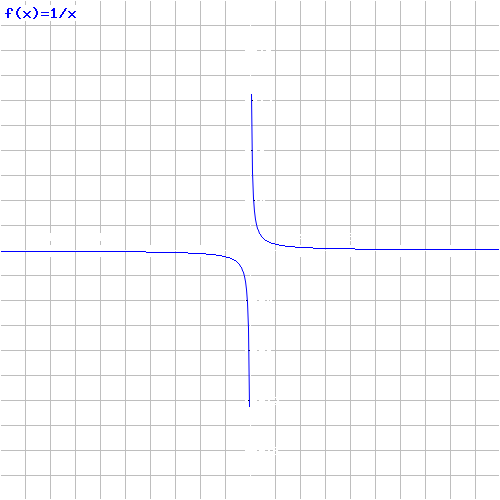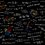# Problems With 0The 0 is a curiously and stranger number. We can't divide by 0, 0! is 1, any number raised by 0 is 1, no matter if the number is the 0 itself.

Today I want to talk about divisions involving 0.

Divide by 0 you can do, but the results are catastrophic!

You know that every number multiplied by 0 is 0 right? Ok, look this:

$0\times 0=0\Rightarrow \frac { 0 }{ 0 } =0$

Ok until now all sounds good, but:

$0\times 1=0\Rightarrow \frac { 0 }{ 0 } =1$

$0\times 2=0\Rightarrow \frac { 0 }{ 0 } =2$

$0=1=2$?!?!? This can be more stranger? Yes, can.

There are many bizarre "evidence" that say: "Any number divided by 0 results in infinity".

The first evidence is:

If the number 1 is divided by a much bigger number, the result approaches to 0:

$\frac { 1 }{ 2 } =0.5$

$\frac { 1 }{ 3 } =0.33333...$

$\frac { 1 }{ 10000000000000 } =0,0000000000001$

$\frac { 1 }{{10}^{100000} } ={10}^{-100000}$

Thus: $\frac { 1 }{ \infty } =0$

However if $\frac { 1 }{ \infty } =0$, $\frac { 1 }{ 0} =\infty$. But, have a catastrophe on here:

$\frac {1 }{ 0 } =\infty \Rightarrow 0\times \infty=1$

If all number multiplied by 0 is 0, why $0\times \infty=1$? $0=1$?

What happens if we plot this function in a graph?

$f(x)=\frac { 1 }{ x }$

Look the image above and see a curiously property of the graph:

When the X axis approaches to 0, the Y axis approaches to $\infty$.

The limit $\displaystyle\lim _{ x\rightarrow 0 }{ \frac { 1 }{ x } =\infty }$, have 2 possible solutions: $+\infty$ or $-\infty$, when $0$ is $+0$ or $-0$ respectively.

Seeing only the positive quadrant of the graph $\displaystyle\lim _{ x\rightarrow +0 }{ \frac { 1 }{ x } =+\infty }$

Seeing only the negative quadrant of the graph $\displaystyle\lim _{ x\rightarrow -0 }{ \frac { 1 }{ x } =-\infty }$

But $-\infty$ and $+\infty$ are two different things and $\infty$ is not a number. $+0$ and $-0$ are not necessarily numbers but something that says if the limit is negative or positive because 0 is neutral with respect to its sign.

Then $\frac{x}{0}$ is indeterminable.

The 0 was a very important number in the history of mathematics, created to represent an empty space, where there was no unity at all. Today he is the target of discussions about it and why it is so mysterious, almost as mysterious as the concept of infinity. What do you think?Note by Victor Paes Plinio
7 years, 2 months ago

This discussion board is a place to discuss our Daily Challenges and the math and science related to those challenges. Explanations are more than just a solution — they should explain the steps and thinking strategies that you used to obtain the solution. Comments should further the discussion of math and science.

When posting on Brilliant:

• Use the emojis to react to an explanation, whether you're congratulating a job well done , or just really confused .
• Ask specific questions about the challenge or the steps in somebody's explanation. Well-posed questions can add a lot to the discussion, but posting "I don't understand!" doesn't help anyone.
• Try to contribute something new to the discussion, whether it is an extension, generalization or other idea related to the challenge.

MarkdownAppears as
*italics* or _italics_ italics
**bold** or __bold__ bold
- bulleted- list
• bulleted
• list
1. numbered2. list
1. numbered
2. list
Note: you must add a full line of space before and after lists for them to show up correctly
paragraph 1paragraph 2

paragraph 1

paragraph 2

[example link](https://brilliant.org)example link
> This is a quote
This is a quote
    # I indented these lines
# 4 spaces, and now they show
# up as a code block.

print "hello world"
# I indented these lines
# 4 spaces, and now they show
# up as a code block.

print "hello world"
MathAppears as
Remember to wrap math in $$ ... $$ or $ ... $ to ensure proper formatting.
2 \times 3 $2 \times 3$
2^{34} $2^{34}$
a_{i-1} $a_{i-1}$
\frac{2}{3} $\frac{2}{3}$
\sqrt{2} $\sqrt{2}$
\sum_{i=1}^3 $\sum_{i=1}^3$
\sin \theta $\sin \theta$
\boxed{123} $\boxed{123}$

Sort by:

I've been playing with this and it came out that it's possible ..

- 6 years, 5 months ago